The STDRATE Procedure

Mantel-Haenszel Effect Estimation

Subsections:

In direct standardization, the derived standardized rates and risks in a study population are the weighted average of the stratum-specific rates and risks in the population, respectively, where the weights are given by the population-time for standardized rate and the number of observations for standardized risk in a reference population.

Assuming that an effect, such as rate difference, rate ratio, risk difference, and risk ratio between two populations, is homogeneous across strata, the Mantel-Haenszel estimates of this effect can be constructed from directly standardized rates or risks in the two populations, where the weights are constructed from the stratum-specific population-times for rate and number of observations for risk of the two populations.

That is, for population k, k=1 and 2, the standardized rate and risk are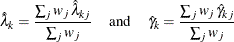where the weights are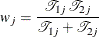for standardized rate, and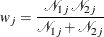for standardized risk.

Rate and Risk Difference Statistics

Denote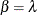for rate and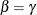for risk. The variance is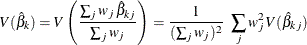The Mantel-Haenszel difference statistic is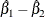with variance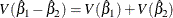Under the null hypothesis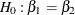, the difference statistic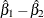has a normal distribution with mean 0.

Rate Ratio Statistic

The Mantel-Haenszel rate ratio statistic is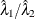, and the log ratio statistic is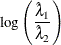Under the null hypothesis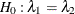(or equivalently,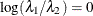), the log ratio statistic has a normal distribution with mean 0 and variance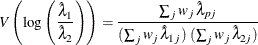where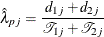is the combined rate estimate in stratum j under the null hypothesis of equal rates (Greenland and Robins 1985; Greenland and Rothman 2008, p. 273).

Risk Ratio Statistic

The Mantel-Haenszel risk ratio statistic is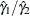, and the log ratio statistic is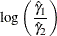Under the null hypothesis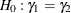(or equivalently,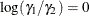), the log ratio statistic has a normal distribution with mean 0 and variance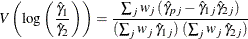where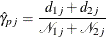is the combined risk estimate in stratum j under the null hypothesis of equal risks (Greenland and Robins 1985; Greenland and Rothman 2008, p. 275).